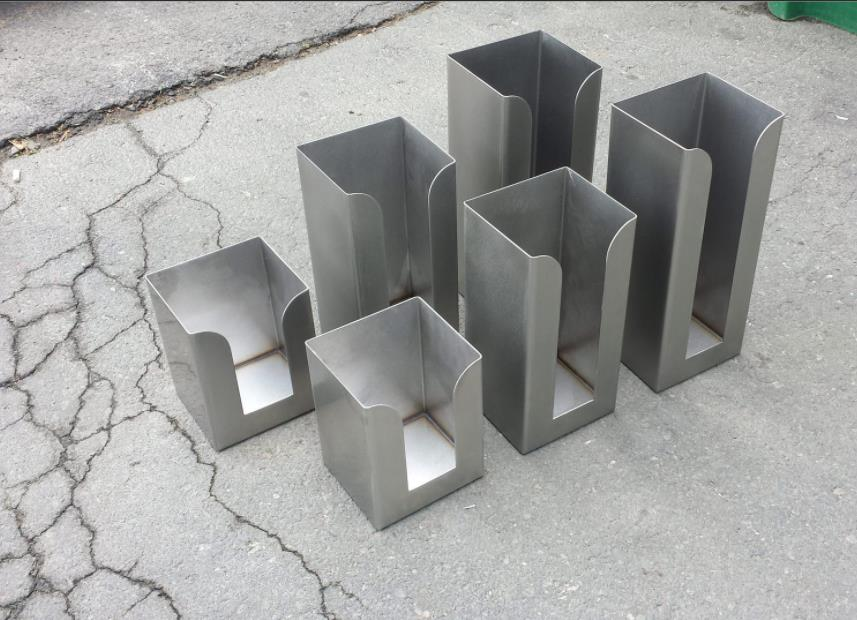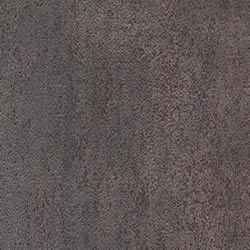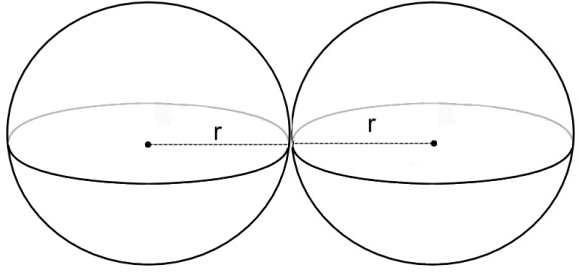# 铁和铁合金的密度

2019-12-0311:47:15铁和铁合金的密度已关闭评论## 铁和铁合金的密度

 铁和铁合金的密度（室温下）材料 密度 克/厘米3 lb m / in 3 纯铁 7.874 0.2845 铸铁 7.866 0.2842 铁艺 7.7 0.2 灰铸铁 7.15 注1 0.258 注1 可锻铁 7.27 注2 0.262 注2 球墨铸铁 7.15 0.258 高镍铁（耐镍） 7.5 0.271 高铬白铁 7.4 0.267

## 铁的物理性质

 铁的物理性质 颜色 银灰色金属 可延展性 能够变形或弯曲 延展性 轻松拉或拉成细线 光泽 有光泽 电导率 良好的热或电传递 同素异形体 它以相同的物理状态以两种或更多种晶体形式出现 拉力 可以拉伸而不会断裂 铁磁 容易磁化

## 铁的化学性质

 铁的化学性质 Ç hemical公式 铁 毒性 无毒 与水反应 与非常热水和蒸汽反应生成氢气 氧化作用 易与潮湿空气中的氧气结合，产生氧化铁，也称为铁锈 溶解度 溶于酸

## 铁的密度公式

7.87g / cm ^ {3}等于7878kg / m ^ {3}

a）如果我们忽略球体之间的空间，则原子的体积为 V = \ frac {M} {\ rho}

V = \ frac {9.27 \ times 10 ^ {-26} kg} {7878kg / m ^ {3}}

V = 1.18 \ times 10 ^ {-29} m ^ {3}

b）为了找到相邻原子的中心之间的距离，我们将使用球体公式的体积，即 \ frac {4 \ pi R ^ {3}} {3}其中R是半径，在我们的例子中是原子的半径。

R = \ left（\ frac {3V} {4 \ pi} \ right）^ {\ frac {1} {3}}

R = \ left（\ frac {3（1.18 \ times 10 ^ {-29} m ^ {3}）} {4 \ pi} \ right）^ {\ frac {1} {3}}

R = 1.41 \ times 10 ^ {-10} m